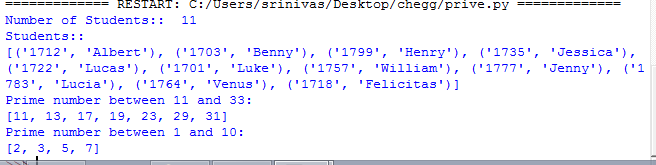# Answered! In this question, write a program testing how the efficiency of double hashing varies when using different prime…

In this question, write a program testing how the efficiency of double hashing varies when using different prime numbers for its hash functions. Double hashing uses two hash functions, one for computing the initial slot and another one for computing the step size of the probe sequence:
hash1(key) = key % prime1 (note: prime1 is the hash table size) hash2(key) = prime2 – key % prime2
In this exercise we investigate how the performance of the hash table changes when using different values for prime1 and prime2.

Task 1: Complete the method readRecords in a pthon file such that it reads a list of student records from the file in the argument and stores them in a list of tuples (StudentID, Name)
Test:
1712 Albert
1703 Benny
1799 Henry
1735 Jessica
1722 Lucas
1701 Luke
1757 William
1777 Jenny
1783 Lucia
1764 Venus
1718 Felicitas

Don't use plagiarized sources. Get Your Custom Essay on
Answered! In this question, write a program testing how the efficiency of double hashing varies when using different prime…
GET AN ESSAY WRITTEN FOR YOU FROM AS LOW AS \$13/PAGE

Output:

Number students: 11

Students: [(1712, ‘Albert’), (1703, ‘ Benny’), (1799, ‘Henry’), (1735, ‘Jessica’)] (etc.)

Task 2: Complete the method primes(m,n) in a python file such that it returns a list of all prime numbers between m and n. If m and/or n are prime numbers they should be included in the list.

Test:
(students.txt)

1712 Albert
1703 Benny
1799 Henry
1735 Jessica
1722 Lucas
1701 Luke
1757 William
1777 Jenny
1783 Lucia
1764 Venus
1718 Felicitas

Output:
Prime number between 11 and 33: [11, 13, 17, 19, 23, 29, 31]

Prime number between 1 and 10: [2, 3, 5, 7]

And there is my code for this question, can anyone help get the correct output?

from HashTable import HashTable

def primes(m,n):
primes_list = []
for number in range(m, n+1):
for i in range(2, number):
if (number % i) == 0:
break
else:
primes_list.append(number)
return primes_list

 def primes(m,n): primes_list = [] for number in range(m, n+1): flag=True for i in range(2, number): if (number % i) == 0: flag=False break if(number!=1 and flag==True): primes_list.append(number) return primes_list def readRecords(filepath): mylist = [] file = open(filepath,’r’) line = file.readlines() file.close() c=0 for i in line: tmp = i.split(‘ ‘) mylist.append((int(tmp),tmp[:len(tmp)-1])) c+=1; print “Number of Students:: “, c return mylist students=readRecords(‘Students.txt’); print “Students::” print students print “Prime number between 11 and 33:” print primes(11,33) print “Prime number between 1 and 10:” print primes(1,10)